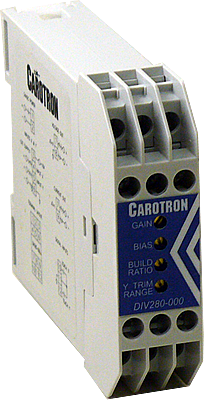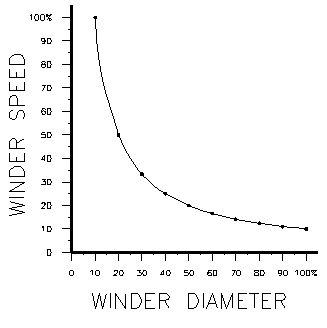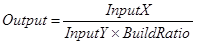#Divider Module DIV280-000

General Description

General DescriptionOne problem encountered in center driven takeup and letoff velocity mode applications is the nonlinear relationship between the diameter of a roll and the motor speed required to maintain constant surface speed of the roll during diameter increase or decrease. A plot of this relationship shows a hyperbolic curve.Figure 1: Winder Speed vs Winder Diameter (10:1 Build Ratio)

When the line speed and roll diameter values are known, the required roll speed can be calculated (i.e. Roll Speed = Line Speed/Roll Diameter). The material surface speed on a takeup or letoff would be held constant during roll diameter changes.

Furthermore, in torque applications, the torque required to maintain constant tension is proportional to roll diameter.  When the line speed and roll rotational speed values are known, the required torque can be calculated (i.e. Roll Diameter = Line Speed/Roll Speed).  The tension applied to the material on a takeup or letoff would be held constant during roll diameter changes.

Model DIV280-000 Voltage Divider Module is designed for the above industrial applications where the division of DC voltage signals is required to produce the non-linear plot.  The module has two voltage inputs (X & Y).  Each voltage input has an associated Teach terminal that can be used to calibrate the minimum and maximum input levels.  The output is based on the following formula:Multiturn GAIN, BIAS, BUILD RATIO, and Y TRIM RANGE potentiometers are used to calibrate the DIV280-000 module.  An internal jumper allows selection of a voltage or current output.  Onboard EEPROM is used to backup and retain the calibration values during a power loss.

VIEW INSTRUCTION MANUAL

View Brochure